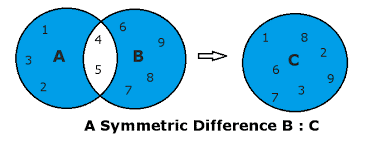# Python Set symmetric_difference: Complete Guide

The symmetric difference of two sets set1 and set2 is the set of elements in either set1 or set2 but not in both.

## Python Set symmetric_difference()

Python set symmetric_difference() is an inbuilt function that returns a new set, the symmetric difference of two sets. For example, if A = {1,2,3,4,5} and B = {4,5,6,7,8,9} are two sets, then the symmetric difference of these two sets is those elements that are present either in Set A or Set B but not in both the sets.Here, we can see that 4 and 5 are present in both the sets, so values except 4 and 5 are symmetric differences of these sets, shown in the set C.

### Syntax

`A.symmetric_difference(B)`

Here A is one set, and B is another set.

### Return Value

This method returns a new set which is the difference between these sets. In addition, it returns a set which is the symmetric difference between the two sets.

See the following code example.

```# app.py

# Declaring two sets

# Even nums between 2 and 10
set1 = {2, 4, 6, 8, 10}
# Multiple of 3 between 1 to 10
set2 = {3, 6, 9}

# priting both the sets
print("Set1 is: ", set1)
print("Set2 is : ", set2)
# Now we will find symmetric difference of these two sets
print("Symmetric difference of set1 and set2 is: ",
set1.symmetric_difference(set2))
```

#### Output

```Set1 is:  {2, 4, 6, 8, 10}
Set2 is :  {9, 3, 6}
Symmetric difference of set1 and set2 is:  {2, 3, 4, 8, 9, 10}
```

Here, we can see that Set1 contains elements of even numbers from 1 to 10, and Set 2 includes elements of multiple of 3 from 1 to 10. So, we can see that 6 is the only value present in both sets. So, according to the definition, except 6, all the values will be the symmetric difference between these two sets.

Let’s see another working example.

```# app.py

list1 = [11, 21, 31]
list2 = [21, 31, 41]
list3 = [31, 41, 51]

# Convert list to sets
set1 = set(list1)
set2 = set(list2)

# Prints the symmetric difference when
# set is passed as a parameter
print(set1.symmetric_difference(set2))

# Prints the symmetric difference when list is
# passed as a parameter by converting it to a set
print(set2.symmetric_difference(list3))```

#### Output

```python3 app.py
{41, 11}
{51, 21}```

We took three lists, converted them into sets, and checked the symmetric difference.

That’s it for this tutorial.

Python set remove()

Python set pop()

Python set issuperset()

Python set issubset()

Python set isdisjoint()

Python set intersection_update()

Python set intersection()